# Generalized envelopes

Generalized envelopes are convenient tool for seismic events detection and their classification and recognition.

For some signal (for instance, sum of channels of seismic array or arrays beam) we can compute its SNR trace (SNR here means signal-to-noise ratio) as follows:

• Compute signals envelope by averaging amplitudes in short time windows (STA: short-time average) say 1 sec;
• Estimate noise level for STA trace;
• Compute signal to noise ratio (SNR).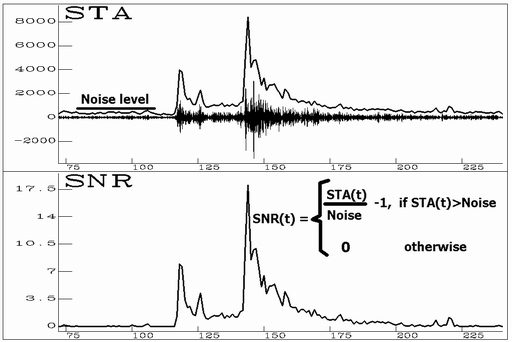Computing SNR trace by STA trace

To compute noise we use an algorithm based on analysis of bar charts of STA trace amplitudes (positive values STAi, i=1,N). First of all we compute maximal value which is still a noise: STAnoise.max after that average amplitudes less than STAnoise.max and consider the result as the noise estimation.

To evaluate STAnoise.max we iteratively compute bar charts of 3 columns from minimal trace amplitude to supposed value of STAnoise.max (for the first iteration we take STAmax), and depending on number of samples in each column (N1,N2,N3) decrease supposed STAnoise.max or consider it to be found and proceed to averaging.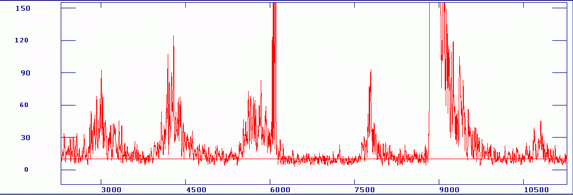Example of noise evaluation. Red line is estimated noise.

For a set of band pass filters fi0-fi1 (f-frequencies) compute SNRi(t) as shown above. Then use SNRi(t) as weights for the frequency bands and compute some weighted functions (i is frequency bands index):

a) Summary SNR: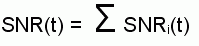b) Weighted frequency: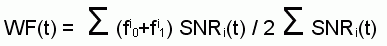SNR(t) shows average behavior of amplitudes in those frequency bands where signal is greater than noise. WF(t) shows change in time of average signal frequency. This couple of functions SNR(t) and WF(t) enables to detect seismic events and often to classify them by comparing with events-samples.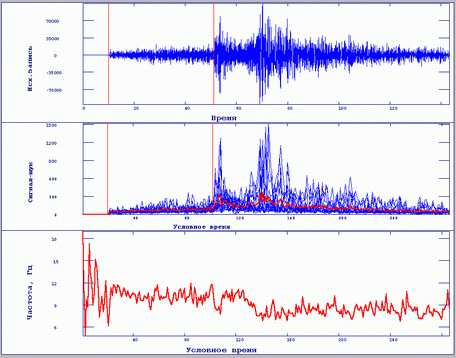Example of computing generalized envelopes. Top plot: source signal. Middle plot: SNRi for different frequency bands (blue) and their average that is summary SNR (red line). Bottom plot: weighted frequency WF(i)

Detecting phases using generalized envelopes.

Introduce functions STA(t) and LTA(t) computed by SNR trace. (This STA differs from those STA which was used to compute SNR trace: there was source signal averaging, here is averaging of SNR trace itself).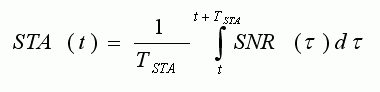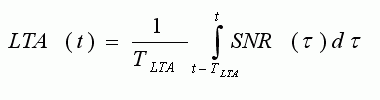For phases detection it is possible to use a function STA(t)-LTA(t). Its maxima where the function is greater than some threshold we consider to be detected phases. Such detector can replace traditional STA/LTA, noise is already taken into account.

Introduce a function (STA-LTA)cumulative which appeared to be useful to select a fragment of recording corresponding to seismic event: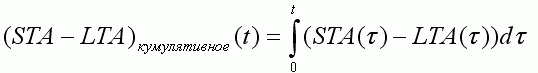We terminate a fragment corresponding a seismic event when (STA-LTA)cumulative crosses 0 (changes its sign from positive to negative).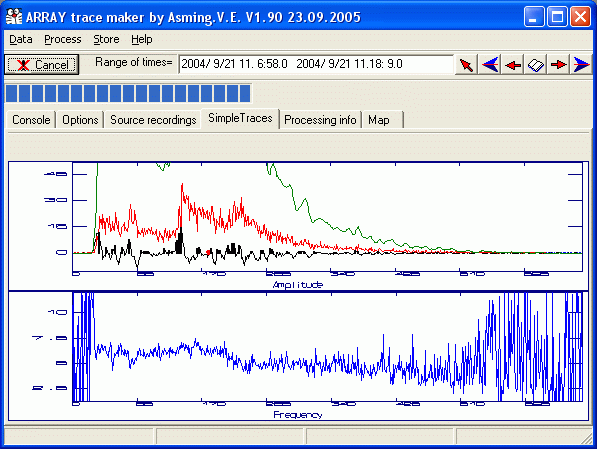Example of events detection by MTR system. Top plot shows SNR(t) trace (red curve), STA(t)-LTA(t) (black curve), detected phases are marked by strokes. Green curve shows (STA-LTA)cumulative which is used to terminate seismic events fragment. A curve on bottom plot (frequency WF(t)) is not used for detection.

Events classification using generalized envelopes.

The functions SNR(t) and WF(t) can be used for comparison of seismic events recordings. To do it it is necessary to reduce them to some fictitious time intervals, containing the same number of samples from P to S for different seismic events.

We computed generalized envelopes for more than 5000 recordings of seismic events made by vertical sensor of Apatity array. Next we tried to compare couples of events by correlation of their SNR traces and difference in WF traces. We used the following measure:

M12 = Corr(SNR1(t),SNR2(t)) - 0.1 <D F12>,
where <D F12> - average difference between WF1 and WF2.

The figures below show our experiments when for some selected event we searched the most similar one using the measure mentioned.Search of similar events for 21.01.1996 earthquake in Northern Norway. A map with the search results and comparison of traces of the source and the most similar events are shown. The most similar event appeared to be in Sweden near Baltic See. The traces are very similar ispite of great distance between the events.Search of similar events for White sea event (probably earthquake). Similar events found are far from the source event and out of mining areas. Many of them are earthquakes in Northern Kereliya and Finland.Search of similar event for Kovdor mining explosion. All similar events found appeared to be explosions! (Olenegorsk, Khibiny, road construction explosion near Polyarnye Zori settlement)

Thus we had shown 3 examples of similar events search:

1. for an event known to be earthquake;
2. for an event which we suspected to be earthquake;
3. for explosion.

For 1st and 2nd cases our system has found very similar events occurred rather far from the source events. Most part of them are out of mining areas. For 3rd case the system has found explosions only although occurred in different mines. We made the same search for many events and discovered that in most cases similar events for explosions are explosions, too. For events occurred far from mining areas the system as usual finds similar ones situated out of mining areas, too, that confims natural origin of the events.

We made an experiment with ARCES recordings of Kirovsk mine (Khibiny Massif) explosions. (15 large underground explosions, 12 large open-pit explosions and 20 small compact underground explosions). We computed generalized envelopes for all of the explosions and for each one searched similar explosions.

For large underground explosions more similar ones appeared to be underground explosions too, for open-pit ones more similar explosions were open-pit, too, with one exception. For compact underground explosions similar ones were both compact and large underground explosions.

Thus, this approach probably can distinguish earthquakes and explosions and even explosions of different kinds. For this it is necessary to make a data base of generalized envelopes of seismic events of known origin.